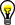# Creating Formula Nodes

## LabVIEW 2018 Help

Edition Date: March 2018
Part Number: 371361R-01
View Product InfoLabVIEW 2016 HelpLabVIEW 2017 HelpLabVIEW 2018 HelpLabVIEW 2019 HelpLabVIEW 2020 Help

Complete the following steps to create a Formula Node.

1. Add a Formula Node to the block diagram.

2. Review the available functions and operators you can use.
3. Use the Labeling tool or the Operating tool to enter the equations you want to calculate inside the Formula Node. Each assignment must have only a single variable on the left side of the assignment (=). Each assignment must end with a semicolon (;). Confirm that you are using the correct Formula Node syntax.

If a syntax error occurs, click the broken Run button to display the Error list window. LabVIEW marks the syntax error with a # symbol.Tip  Add comments to the text in a Formula Node by enclosing them inside a slash-asterisk pair (/*comment*/), or after a double-slash (//comment).
1. Create an input terminal for each input variable by right-clicking the Formula Node border and selecting Add Input from the shortcut menu. Type the variable name in the terminal that appears. You can edit the variable name at any time using the Labeling tool or the Operating tool, except when the VI is running.

Variable terminals are case sensitive. There is no limit to the number of terminals or equations in a Formula Node. You can change a terminal type or remove a terminal.
2. Create an output terminal for each output variable by right-clicking the Formula Node border and selecting Add Output from the shortcut menu. Type the variable name in the terminal that appears. You can edit the variable name at any time using the Labeling tool or the Operating tool, except when the VI is running. Output variables have thicker borders than input variables.Note  No two inputs and no two outputs can have the same name. However, an output can have the same name as an input.
1. (Optional) The default data type for output terminals is double-precision, floating-point. To change the data type, create an input terminal with exactly the same name as the output terminal and wire a data type to that input terminal. Doing so also provides a default value for the terminal. You also can use the Formula Node syntax to define the variable inside the Formula Node. For example, int32 y; changes the data type of the output terminal y to 32-bit integer.
2. Wire the input and output terminals of the Formula Node to their corresponding terminals on the block diagram. All input terminals must be wired. Output terminals do not have to be wired.